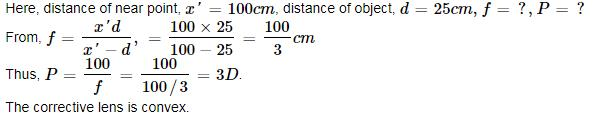Courses

# Test: Optical Instruments

## 10 Questions MCQ Test Physics Class 12 | Test: Optical Instruments

Description
This mock test of Test: Optical Instruments for Class 12 helps you for every Class 12 entrance exam. This contains 10 Multiple Choice Questions for Class 12 Test: Optical Instruments (mcq) to study with solutions a complete question bank. The solved questions answers in this Test: Optical Instruments quiz give you a good mix of easy questions and tough questions. Class 12 students definitely take this Test: Optical Instruments exercise for a better result in the exam. You can find other Test: Optical Instruments extra questions, long questions & short questions for Class 12 on EduRev as well by searching above.
QUESTION: 1

### A person cannot see the object distinctly, when placed at a distance less than 100cm. What is the power of the spectacles that he should use to see clearly the objects placed at 25 cm?​

Solution:QUESTION: 2

### An astronomical telescope has a large aperture to:

Solution:

The aperture of an astronomical telescope is defined as the diameter of the objective lens.
In telescopes since the stars are very far away from us and emit light intensities, we need a large aperture to increase the amount of light entering the telescope thereby increasing the resolution.

QUESTION: 3

### Resolving power of a telescope depends on

Solution:

The resolving power of a telescope is given by RP∝2μsinθ​/122λ
Further, sinθ ∝ tanθ ∝ diameter of the objective.
So, the resolving power of a telescope depends on the diameter of the objective lens.

QUESTION: 4

A far- sighted person has lost his spectacles. He can read a book at usual distance by looking through a pin hole because while looking through the pin hole:

Solution:

Long-sighted people who have lost their spectacles can still read a book by looking through a small (3-4 mm) hole in a sheet of paper because by doing so due to the diffraction at the hole the focal length of the eye lens is effectively decreased. Hence the correct option is C.

QUESTION: 5

A myopic person can see things clearly if they lie between 8 cm and 100 cm from his eye. The lens will enable him to see the moon distinctly if its focal length is:​

Solution:

The final image of the moon should be formed at a distance of 100 cm. Using a concave lens of focal length 100 cm will enable him  to see the moon distinctly.

Focal length of lens =-100 cm

QUESTION: 6

The earth takes 24 h to rotate once about its axis. How much time does the sun take to shift by 1º when viewed from the earth?​

Solution:

Time taken for 360° shift = 24 h
Time taken for 1° shift = 24/360 h = 4 min.

QUESTION: 7

A slide projector gives a magnification of 10. If a slide of dimensions 3 cm x 2 cm is projected on the screen, the area of the image on the screen is:​

Solution:

Area of magnification,
A1​A22
A1/6​​=100
A1​=600 cm2

QUESTION: 8

What happens to the magnifying power of microscope, when its length increases?​

Solution:

Magnification in microscope is  m=− lD/f0​​fe
where, l is length of tube and D is least distance for clear vision
f0​ is focal length of objective
fe​ is the focal length of the eyepiece.
So, as l increases m increases.
Hence, the magnifying power of a microscope increases with increase in tube length.

QUESTION: 9

The magnifying power of a telescope is 9. When it is adjusted for parallel rays, the distance between the objective and eyepiece is found to be 20 cm. The focal lengths of lenses are:​

Solution:

f0/fe ​​=9,         ∴f0​=9fe
Also f0​+fe​=20             (∵ final image is at infinity)
9fe​+fe​=20,fe​=2cm,
∴f0​=18cm

QUESTION: 10

The distance of distinct vision of a person is 50 cm. He wants to read a book placed at 25 cm. What should be the focal length of the spectacles?​

Solution:

'u' should be the least distance of distinct vision.
U=-25cm
V=-50
(1/f)=(1/v)-(1/u)
=(-1/50) + (1/25)
=1/50
F=50cm
Hence a convex lens of focal length 50cm should be used.